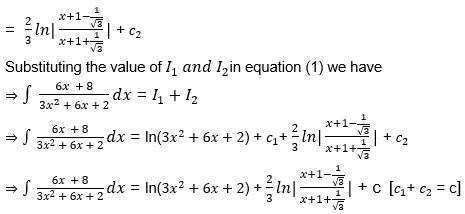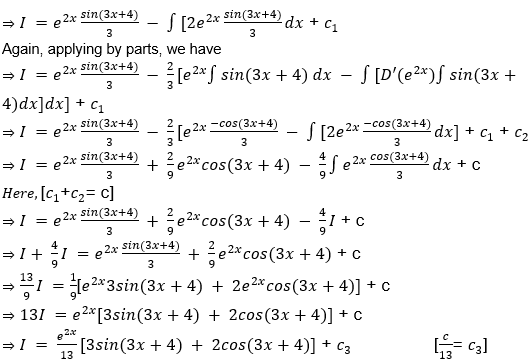# Indefinite Integrals Problems and Solutions

In calculus, Integration is defined as the inverse process of differentiation and hence the evaluation of an integral is called as anti derivative. It is a process of the summation of a product. If we have not said the summation is to be done from which point to which point. In other words, the interval of summation is indefinite and hence these types of integrals are known as indefinite integrals. Integration is an important part of calculus. It includes single integral, double integral, and multiple integrals. Various types of integral are used to find surface area and the volume of geometric solids.

## What is Infinite Integral in Calculus?

The indefinite integral is defined as a function that will describe an area under the function’s curve from an undefined point to another arbitrary point. The absence of a specified first point leads to an arbitrary constant most often denoted as C and is always considered a part of indefinite integral.

In other words, the indefinite integral is the family of all functions whose derivative is f(x) but with a possibly finite set of exceptions. $\frac{d}{dx}\int f(x)dx = f(x)$.

Indefinite integrals are integrals without limits. The technique of integration is very useful in two ways.

• To find the function whose derivative is given.
• To find the area bounded by a curve given by the function under certain conditions.

### Rules of Integration

The anti derivative of a definite integral is only implicit, that is, the solution will only be in a functional form. That is, ∫f(x)dx = g(x) + C, where g(x) is another function of x and C is an arbitrary constant. However, there are many integrals which are to be integrated within a given interval. They are denoted in general as $\int_a^b$f(x)dx where, a and b are the limits of the interval. Such types of integrals are known as definite integrals. The solution of a definite integral is unique and the solution to $\int_a^b$f(x)dx is F(b) – F(a), where F(x) is the anti derivative of the given integral.

There are few important rules for integration

Integration of some functions may be readily done for functions whose derivatives are known. But, in many cases, the integrant (the function to be integrated) may not be that simple. It may be a sum, difference, product or a quotient of two functions. To perform the integration of such functions we need to follow the basic integration rules. Some basic integration rules as given below.

Rule 1: The integration of a sum or difference of two functions is the sum or difference (respectively) of the integration of the individual functions. That is,

∫[f(x) + g(x)]dx = ∫f(x)dx + ∫g(x)dx

Rule 2: The integration of a product of a function is [ First function * the integration of the second function – integration of { the integration of the second function*derivative of the first function}]

That is, if u and v are two functions, ∫udv = uv − ∫vdu

For a better memory aid, we had chosen dv as the second function. Hence, in all the integration of the product of two functions, we equate the second function as dv and immediately figure out what is v. Also you should know the technique of correctly assigning the first and second functions.

There are many ways to find the integration of a given function such as:

• Integration by Parts
• Integration by Substitution Method or Change of Variable
• Directly use the formula
• Integration by Partial Fraction Method

## Solved Problems on Indefinite Integrals for JEE

Practice below problems to crack your exam.

Question 1: Solve ∫(x2 + 3x – 2)dx

Solution: ∫(x+ 3x – 2)dx = (x3/3)+ (3x2)/2 – 2x + c.

Question 2: Solve ∫ 4x e2x dx

Solution: ∫ 4x e2x dx = ∫ 22x  e2xdx = ∫ (2e)2x dx =(2e)2x/(2 +log 4) + c

Question 3: $\int \frac{1 – tan^2 x}{1 + tan^2 x}$ dx

Solution:

$\int \frac{1 – tan^2 x}{1 + tan^2 x}$ dx

= $\int \frac{1 – tan^2 x}{sec^2 x}$ dx

= $\int (\frac{1}{sec^2 x} – \frac{tan^2 x}{sec^2 x}$ dx

= $\int (cos^2 x – sin^2 x) dx$

= $\int cos2 x dx$

= $\frac{sin 2x}{2} + C$

Question 4: Solve $\int \frac{1}{1+cos 6 x}$ dx

Solution:

$\int \frac{1}{1+cos 6 x}$ dx

= $\int \frac{1}{2cos^2 3 x}$ dx

= $\int \frac{1}{2} sec^2 3x$ dx

= tan(3x)/6 + C

Question 5: Solve $\int \frac{x^4}{1+x^{10}}$

Solution: $\int \frac{x^4}{1+x^{10}}$

= $\int \frac{x^4}{1+(x^5)^2}$

Let us use substitution method to solve the problem.

Put x5 = t, then

Differentiating it we have

⇒ 5x4 dx = dt

⇒ x4 dx = dt/5

Putting this value in equation (1) we have

=> $\int \frac{1}{5(1+t^2)}= 1/5 \times tan^{-1} t + C$

=> (1/5) tan-1 x5 + C

Question 6: Evaluate $\int \frac{m^2 tan^{-1}m^3}{1+m^6} dm$

Solution:

$\int \frac{m^2 tan^{-1}m^3}{1+m^6} dm$

= $\int \frac{m^2 tan^{-1}m^3}{1+(m^3)^2} dm$ …(1)

Let tan-1 m3 = t

Differentiating it we have

⇒ 3m2/(1 + m6 ) dm = dt

⇒ m2(1 + m6 ) dm = dt/3

Substituting this value in the equation (1) we have

$\int \frac{m^2 tan^{-1}m^3}{1+(m^3)^2} dm = \int \frac{t}{3}dt$

= t2/2(3)  + C

= $\int \frac{(tan^{-1}m^3)^2}{6}+C$

Question 7: Solve $\int \frac{sin 8x}{1-cos^4 4x} dx$

Solution: $\int \frac{sin 8x}{1-cos^4 4x} dx$

= $\int \frac{sin 8x}{\sqrt{(1 – (cos^2 4x)^2 }}$ dx (1)

Let cos2 4x = t

Differentiating it we have

⇒ – 2cos(4x).sin(4x) dx = dt

⇒ – sin(8x) dx = dt

⇒ sin(8x) dx = -dt

Substituting this value in the equation (1) we have

$\int \frac{sin 8x}{\sqrt{(1 – (cos^2 4x)^2 }}$ dx

= $\int \frac{-1}{\sqrt{(1 -t^2 }}$ dt

= -sin-1 t + c

= -sin-1 cos2 4x + c.

Question 8: Solve $\int \frac{6x+8}{3x^2+6x+2}$ dx

Solution:

$\int \frac{6x+8}{3x^2+6x+2}$ dx

= $\int \frac{AD’(3x^2+6x+2)}{3x^2+6x+2} dx + \int \frac{B}{3x^2+6x+2} dx$

⇒ D’(3x2+6x+2) = 6x + 6

So, 6x + 8 = A(6x + 6) + B

By comparing coefficients we have

⇒ A = 1 and B = 2

So we have

$\int \frac{6x+8}{3x^2+6x+2} =\int \frac{6x+6}{3x^2+6x+2}dx + \int \frac{2}{3x^2+6x+2}dx$

= I1 + I2 …(1)

Where I1 = $\int \frac{6x+6}{3x^2+6x+2}dx$ …(2) and

I2 = = $\frac{2}{3x^2+6x+2}dx$

Solve for I1

Let 3x2+6x+2 = t

Differentiating it we have

⇒ (6x + 6) dx = dt

Substituting this value in equation (2) we have

$I_1 = \int \frac{1}{t} dt = ln(t) + c = ln(3x^2+6x+2) + c_1$

And

$I_2 = \int \frac{2}{3x^2+6x+2} dx = \int \frac{2}{3(x^2+2x+\frac{2}{3}}dx$

= $\int \frac{2}{3(x+1)^2 – 1 + \frac{2}{3}}dx$

= $\int \frac{2}{3(x+1)^2 – \frac{1}{3}}dx$Question 9: Solve ∫ e2x cos(3x + 4) dx

Solution:

Let I = ∫ e2x cos(3x+4) dx

Taking e2x as first and cos(3x + 4) as the second function and applying integration by parts we have

⇒ I = ∫ e2x cos(3x+4) dx

⇒ I = e2x ∫ cos(3x+4) dx – ∫[D'(e2x)∫ cos(3x+4)dx]dxQuestion 10: Solve the integral, $sin(ln y) + cos(ln y)] dy$

Solution:

$sin(ln y) + cos(ln y)] dy$

Let I = ∫[sin(ln y) + cos(ln y)] dy….(1)

Let, ln y = t

⇒ y = et

Differentiating it we have

⇒ dy = edt

Substituting in equation (1) we have

⇒ I = ∫ e[sin(t) + cos(t)] dt

D’(sin(t)) = cos(t)

Using the result ∫ ex [f(x) + f’(x)] dx = ex  f(x) + c, we can say

⇒ I=∫ e[sin(t) + cos(t)] dt = esin(t) + c

⇒ I = e(ln y)sin(ln y) + c

⇒ I = y. sin(ln y) + c .

#### Indefinite Integration – Video Lesson#### Indefinite-Integration Problems